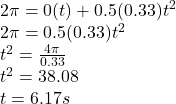## ‏A 50 – N x m torque acts on a wheel with a moment of inertia 150 kg x m² . If the wheel starts from rest , how long will it take the wheel

Question

‏A 50 – N x m torque acts on a wheel with a moment of inertia 150 kg x m² . If the wheel starts from rest , how long will it take the wheel to make one revolution ?

in progress 0
5 months 2021-08-26T05:46:47+00:00 1 Answers 25 views 0

## Answers ( )

t = 6.17 s

Explanation:

For a 1 revolution movement,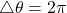Torque,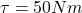Moment of Inertia,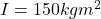If the wheel starts from rest,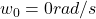The angular displacement of the wheel can be given by the formula: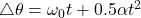…………….(1)

Where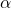is the angular acceleration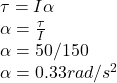To get t, put all necessary parameters into equation (1)